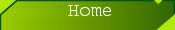# Exponential Random Variable

Definition 6.1-1

A random variable X is said to have an exponential distribution if the probability density function is given by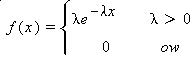The following graph illustrates how the exponential curve changes for different values of l.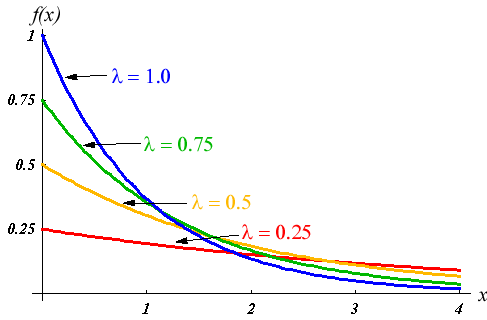For any positive integer n,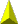Theorem 6.1-1:

The mean, variance, and standard deviation of an exponential random variable X are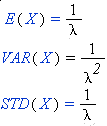The cumulative distribution function isExample 1:

Assume that the amount of time it takes to complete a customer's transaction at an ATM machine is an exponential random variable with mean of 2 minutes. This means that l=2. So,

E(X) = 1/ l =1/2

VAR(X) = 1/ l^2 =1/4

STD(X) =1/ l= 1/2

The probability that it takes less than 3 minutes to complete a transaction is

P(X<3) = P(X£ 2)=F(2)=1-exp(-2*2)= 0.982

The probability that it takes more than 4 minutes to complete a transaction is

P(X>4)=1-P(X £ 4)= 1- F(4) = 1- [ 1-exp(-2*4)]=exp(-2*4)=0.000335Example 2:

Assume that the amount of time it takes to process your order at an ice cream store is an exponential random variable with mean of 2.2 minutes.

 What is l? (Round to 2 decimal places) What is E(X) ? (Round to 2 decimal places) What is VAR(X)? (Round to 2 decimal places) What is STD(X)? (Round to 2 decimal places) What is P(X£2)? (Round to 4 decimal places) What is P(X>3) ? (Round to 4 decimal places)

SolutionExample 3:

Let X and Y be independent exponential random variables with E(X)=3 and E(Y) =4.6

E(X+Y)= E(X)+ E(Y)=3+4.6= 7.6

VAR(X+Y)= VAR(X)+ VAR(Y)=(3)^2 +(4.6)^2= 9+21.16=30.16

E(X)=1/l =3 implies that l=1/3 so f(x) =(1/3) exp(-x/3)

E(Y)=1/l =4.6 implies that l= 1 / 4.6 so f(y)=(1/4.6) exp(-y/4.6)

Since X and Y are independent, f(x,y) can be found simply by multiplying f(x) and f(y).So,

f(x,y)= (1/3) exp(-x/3) * (1/4.6) exp(-y/4.6)= (1/13.8) exp(-x / 3) exp(-y / 4.6)

where 0<x<¥ and 0<y<¥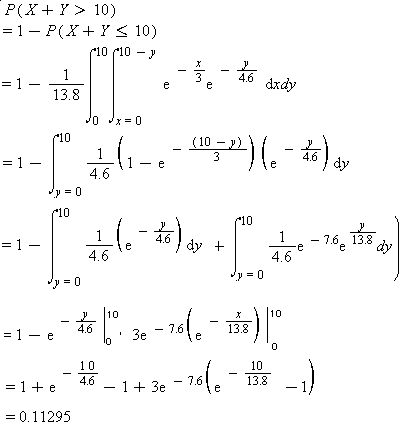Example 4:

Let X and Y be independent exponential random variables with E(X)=4 and E(Y) =5.1

 What is E(X+Y)? What is VAR(X+Y)? (Round to 2 decimal places) Find f(x). What is the value of l? (Round to 2 decimal places) Find f(y).What is the value of l? (Round to 2 decimal places)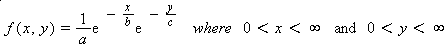Answer the following questions using the defintion of f(x,y) given above.

 What is the value of a? What is the value of b? What is the value of c? What is P(X+Y >8)? (Round to 4 decim al places)

Solution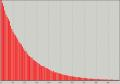Use the following applet to explore the Exponential Distribution!Help!## Useful Web Resources

Engineering Statistics HandbookSolutions

Solution to Example 2

l=2.2

E(X) = 1/ l =1/2.2 =0.45

VAR(X) = 1/ l^2 =1/(2.2)^2=0.21

STD(X)=1/ l= 0.458= 0.46

The probability that it takes less than or equal to 2 minutes to process your order is

P(X£ 2)=F(2)=1-exp(-2.2*2)= 0.9877

The probability that it takes more than 3 minutes to process your order is

P(X>3)=1-P(X£3)= 1- F(3) = 1- [ 1-exp(-2.2*3)]=exp(-2.2*3)=0.0014Solution to Example 4

E(X)=4 and E(Y) =5.1

E(X+Y)= E(X)+ E(Y)= 4+5.1= 9.1

VAR(X+Y)= VAR(X)+ VAR(Y)=(4)^2 +(5.1)^2= 42.01

Find the joint probability distribution of f (x,y):

E(X)=1/l =4 implies that l=1/4 =0.25

So f(x) =(1/4) exp(-x/4) and

E(Y)=1/l =5.1 implies that l= 1 / 5.1 =0.196=0.20

So f(y)=(1/5.1) exp(-y / 5.1)

Since X and Y are independent, f(x,y) can be found simply by multiplying f(x) and f(y). So,

f(x,y) = (1/4) exp(-x / 4) * (1/ 5.1) exp(-y / 5.1)= (1/20.4) exp(-x / 4) exp(-y / 5.1) where 0<x<¥ and 0<y<¥

So a= 20.4 , b=4 and c=5.1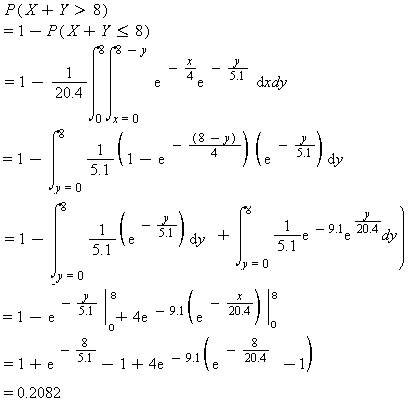Help!

When the Web brower is opened, scroll to the bottom of the page until you find a drop down box that says Select Applet. Choose Exponential Probabilities and click Open!. When the applet opens you can enter in values for l, X0 and/or X1. Press Calculate! and the probability, pdf function and cdf function will be displayed on your screen. Enjoy!Created by Ranodya Upathilake © Copyright 2002-2003. All rights reserved. Free Web Site Templates by JimWorld.com Designed by Finerdesign.com | Comments or Questions?